Home > A2C > Chapter 7 > Lesson 7.2.2 > Problem7-121

7-121.
1. Which of the following statements are true? If true, explain how you know, and if not, show why not. Homework Help ✎

1.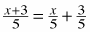2.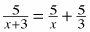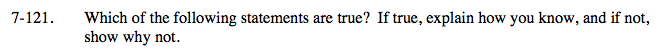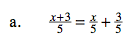Try multiplying both sides by 5.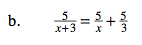Is there something you can multiply both sides by to eliminate the fractions?

The equation is only true when

$\textit{x}=\frac{-3\pm3\textit{i}\sqrt{3}}{2}$